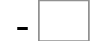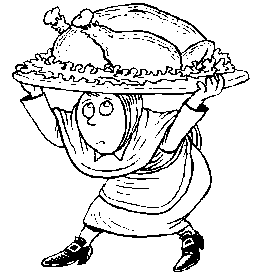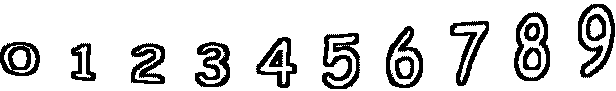10= 0   0= 04= 4   4= 3   0= 0   10= 7 9= 3   7= 6   7= 4   0= 0